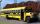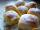# Pupils

Two classes to collect money. Boys are four sevenths pupils. In time did not pay a quarter of the boys and a sixth of girls, which together mean 12 sinners. How many pupils attend this two classes ?

Result

x =  56

#### Solution:

4/7*1/4*x + (1-4/7)*1/6* x = 12

18x = 1008

x = 56

Calculated by our simple equation calculator.

Leave us a comment of example and its solution (i.e. if it is still somewhat unclear...):

Showing 0 comments:Be the first to comment!#### To solve this example are needed these knowledge from mathematics:

Need help calculate sum, simplify or multiply fractions? Try our fraction calculator. Do you have a linear equation or system of equations and looking for its solution? Or do you have quadratic equation?

## Next similar examples:

1. SchoolThere are 150 pupils in grade 5 . 2/3 of it are female. By what fractions are the males?
2. Lengths of the poolMiguel swam 6 lengths of the pool. Mat swam 3 times as far as Miguel. Lionel swam 1/3 as far as Miguel. How many lengths did mat swim?
3. Roman numerals +Add up the number writtens in Roman numerals. Write the results as a decimal number.
4. Eq1Solve equation: 4(a-3)=3(2a-5)
5. Addition of Roman numbersAdded together and write as decimal number: LXVII + MLXIV
6. Adding mixed numbersAdd this two mixed numbers: 1 5/6 + 2 2/11=
7. Flood waterFlood waters in some US village meant that the homes had to evacuate 364 people. 50 of them stayed at elementary schools, 59 them slept with their friends and others went to relatives. How many people have gone to relatives?
8. CookiesMom bake cookies. Rolo took 2/9 of all cookies, Michal 3/9. How many cookies ate Rolo if Michal had 9.
9. Roman numerals 2+Add up the number writtens in Roman numerals. Write the results as a roman numbers.
10. Forest nurseryIn the forest nursery after winter, they found that 1/10 stems died out of them. For them, they land 193 new spruces. How many spruces are in the forest nursery?
11. Cakes1/3 poppy cake, 1/3 apple, 15 pieces of cheese. How many are totally cakes?
12. Simple equation 5Solve equation with fractions: X × 3/8 = 1/2
13. DriveAt the bus stop 8 people take out and 10 people take into bus. Next stop 6 take out and 5 take in. On the third stop 6 take out and 3 take in. The bus traveled further with 39 people. How many passengers were originally at the bus?
14. Addition of mixed numeralsAdd two mixed fractions: 2 4/6 + 1 3/6
15. Jam cakesMom baked a third of plum jam cakes, one third cheesecakes and 18 poppy. How many cakes she had bake?
16. ZdeněkZdeněk picked up 15 l of water from a 100-liter full-water barrel. Write a fraction of what part of Zdeněk's water he picked.
17. In fractionsAn ant climbs 2/5 of the pole on the first hour and climbs 1/4 of the pole on the next hour. What part of the pole does the ant climb in two hours?